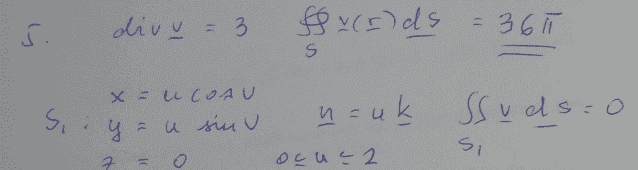# Surface integral over a cylinder

• faen

## Homework Statement

Here's a picture of the question:Here's the solution:## The Attempt at a Solution

I can't really make complete sense of some things around this... Like how did the integral become 36pi based on what is written in the solution? Why is z equal to 0.. What is the double integral over surface S1 and why is it zero? How is the divergence 3 if z is zero? Also if i use stokes theorem and find the volum integral, it would equal to 3 times the volume of the sylinder since the divergence is 3. But it doesn't equal to 36 pi... So if somebody could enlighten me around these questions i'd really appreciate it :)

the problem is solved, so no more help is required.